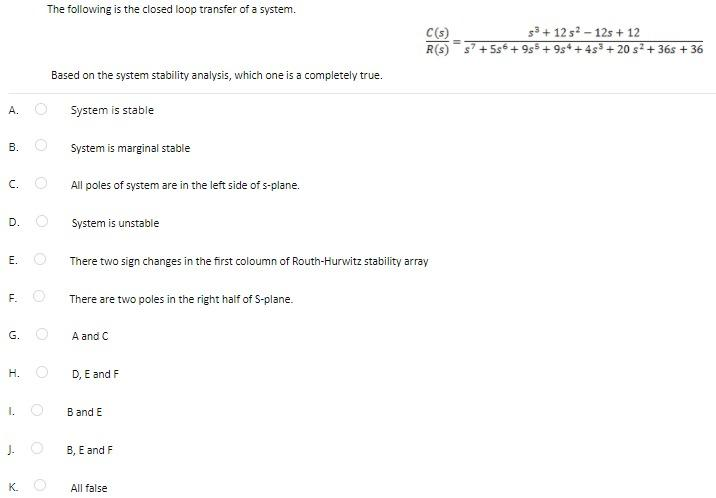# Question The following is the closed loop transfer of a system. C(3) R(S) 33+ 12 s2 - 12s + 12 +995 +98 +48 + 20 s+ 365 +36 Based on the system stability analysis, which one is a completely true. A. System is stable B. System is marginal stable c. All poles of system are in the left side of s-plane. D. System is unstable E. There two sign changes in the first coloumn of Routh-Hurwitz stability array F. There are two poles in the right half of 5-plane. G. A and C H. D, E and F 1. Band E J. B, E and F K. All falseFPC6AR The Asker · Electrical EngineeringTranscribed Image Text: The following is the closed loop transfer of a system. C(3) R(S) 33+ 12 s2 - 12s + 12 +995 +98 +48 + 20 s+ 365 +36 Based on the system stability analysis, which one is a completely true. A. System is stable B. System is marginal stable c. All poles of system are in the left side of s-plane. D. System is unstable E. There two sign changes in the first coloumn of Routh-Hurwitz stability array F. There are two poles in the right half of 5-plane. G. A and C H. D, E and F 1. Band E J. B, E and F K. All false
More
Transcribed Image Text: The following is the closed loop transfer of a system. C(3) R(S) 33+ 12 s2 - 12s + 12 +995 +98 +48 + 20 s+ 365 +36 Based on the system stability analysis, which one is a completely true. A. System is stable B. System is marginal stable c. All poles of system are in the left side of s-plane. D. System is unstable E. There two sign changes in the first coloumn of Routh-Hurwitz stability array F. There are two poles in the right half of 5-plane. G. A and C H. D, E and F 1. Band E J. B, E and F K. All false